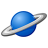# App Development

Discussion board where members can learn more about Qlik Sense App Development and Usage.

Announcements
Welcome to Qlik Community! Check out our new navigation! FIND OUT MORE
cancel
Showing results for
Did you mean:Contributor II

## Cumulated sum (loops in expression?)

Hi all,

So I'm wondering if its possible to do a loop within an expression in QlikSense.

I've got income data across 2 years, and want to do YTD income comparisons - so if i'm in March 2017, for 2016, I need to add up Jan/Feb/March but ignore the rest of the year..

I can do this with a lengthy set of IF statements: (vMonth & vYear are user controllable variables from the report)

eg:

---------------------------------------------------------------------

//January

if(\$(vMonth)>= 1,

//In Month

Sum(if(monthstart(cashbookdate) = Makedate(\$(vYear)-1,1)

and (EffectiveDate <=  MonthEnd(MakeDate(\$(vYear)-1,1)) or (isnull(EffectiveDate) and year(cashbookdate) = \$(vYear)-1))

and TranType = 'New Business'

,   (Commission_Calcd)))

+

//Brought forward

Sum(if(cashbookdate < MakeDate(\$(vYear)-1, 1)

and MonthStart(EffectiveDate) = MakeDate(\$(vYear)-1, 1)

and TranType = 'New Business'

,   (Commission_Calcd)))

,0)

+

//February

if(\$(vMonth)>= 2,

//In Month

Sum(if(monthstart(cashbookdate) = Makedate(\$(vYear)-1,2)

and (EffectiveDate <=  MonthEnd(MakeDate(\$(vYear)-1,2)) or (isnull(EffectiveDate) and year(cashbookdate) = \$(vYear)-1))

and TranType = 'New Business'

,   (Commission_Calcd)))

+

//Brought forward

Sum(if(cashbookdate < MakeDate(\$(vYear)-1, 2)

and MonthStart(EffectiveDate) = MakeDate(\$(vYear)-1, 2)

and TranType = 'New Business'

,   (Commission_Calcd)))

,0)

+

//March

---------------------------------------------------------------------

//Continued for the rest of the months..

but this takes a very long time for Qlik to calculate - and also, when trying to do YOY % this means 2 lots of that code (current year / prior year) which doesnt actually fit in the allowed space in QlikSense as a formula.

I was hoping to do something like:

---------------------------------------------------------------------

Set i = 1;

do while \$(vMonth) >= i

Sum(if(monthstart(cashbookdate) = Makedate(\$(vYear),i)

and (EffectiveDate <=  MonthEnd(MakeDate(\$(vYear),i)) or (isnull(EffectiveDate) and year(cashbookdate) = \$(vYear)))

and TranType = 'New Business'

,   (Commission_Calcd)))

+

//Brought forward

Sum(if(cashbookdate < MakeDate(\$(vYear), i)

and MonthStart(EffectiveDate) = MakeDate(\$(vYear), i)

and TranType = 'New Business'

,   (Commission_Calcd)))

Let i = i + 1;

loop

---------------------------------------------------------------------

but Sense doesnt seem to like loops in the expressions? At least, not how I've typed it there anyway.

Does anyone know of any way I could add up the calculations above in as little code as possible to get away from the list of IF statements?

Thanks,

Alec

Labels (1)
• ### expression

4 RepliesMVP

You might be able to use ValueLoop here, but difficult to give an exact expression without testing it out.

Missing Manual - ValueLoop() &amp; ValueList()Contributor II
Author

Thanks for the reply Sunny, this looks promising - can I use multiple ValueLoops like below?

Sum(if(\$(vMonth)>= valueloop(1,12)),

//In Month

Sum(if(monthstart(cashbookdate) = Makedate(\$(vYear),valueloop(1,12))

and (EffectiveDate <=  MonthEnd(MakeDate(\$(vYear),valueloop(1,12))) or (isnull(EffectiveDate) and year(cashbookdate) = \$(vYear)))

and TranType = 'New Business'

,   (Commission_Calcd)))

+

//Brought forward

Sum(if(cashbookdate < MakeDate(\$(vYear), valueloop(1,12))

and MonthStart(EffectiveDate) = MakeDate(\$(vYear), valueloop(1,12)

and TranType = 'New Business'

,   (Commission_Calcd)))))

The expression box says its fine but it returns nothing..MVP & Luminary

If your LYTD/YTD period is fix to today it's quite common to create flags for them within a master-calendar. Are they instead variable you will need a different approach by creating a PeriodID within the sorted master-calendar, for example:

autonumber(Year & '|' & Month) as PeriodID

and then you could use this PeriodID within a set analysis condition like:

sum({< PeriodID = {">=\$(=max(PeriodID)-12)<=\$(=max(PeriodID))"}>} Value)

sum({< PeriodID = {">=\$(=max(PeriodID)-24)<=\$(=max(PeriodID)-12)"}>} Value

More to master-calendars and various calculations with periods could you find here: How to use - Master-Calendar and Date-Values.

- MarcusMVP

Like I said, it would be difficult to give much suggestion before have a look at this. May be there is an easier way to do this without using loops (something like marcus_sommer‌'s suggestion), but without seeing a sample it would be difficult to comment.Tags
Community Browser